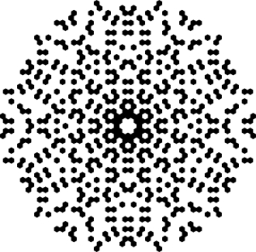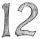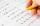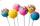# Lcm = 22 + gcd

The least common multiple of two numbers is 22 more than their greatest common divisor. Find these numbers.

a =  5

### Step-by-step explanation:Did you find an error or inaccuracy? Feel free to write us. Thank you!Tips to related online calculators
Do you want to calculate least common multiple two or more numbers?
Do you want to calculate greatest common divisor two or more numbers?

## Related math problems and questions:

• Gcd and lcmCalculate the greatest common divisor and the least common multiple of numbers. a) 16 and 18 b) 24 and 22 c) 45 and 60 d) 36 and 30
• LCD 2The least common denominator of 2/5, 1/2, and 3/4
• MO C–I–1 2018An unknown number is divisible by just four numbers from the set {6, 15, 20, 21, 70}. Determine which ones.
• The ticketsThe tickets to the show cost some integer number greater than 1. Also, the sum of the price of the children's and adult tickets, as well as their product, was the power of the prime number. Find all possible ticket prices.
• DecomposeDecompose into primes and find the smallest common multiple n of (16,20) and the largest common divisor D of the pair of numbers (140,100)
• School booksAt the beginning of the school year, the teacher distributed 480 workbooks and 220 textbooks. How many pupils could have the most in the classroom?
• Apples and pearsMom divided 24 apples and 15 pears to children. Each child received the same number of apples and pears - same number as his siblings. How many apples (j=?) and pears (h=?) received each child?
• Length of a stringWhat is the smallest length of a string that we can cut into 18 equal parts and even 27 equal parts (in decimeters)?
• 9.A9.A to attend more than 20 students but fewer than 40 students. A third of the pupils wrote a math test to mark 1, the sixth to mark 2, the ninth to mark 3. No one gets mark 4. How many students of class 9.A wrote a test to mark 5?
• Common divisorsFind all common divisors of numbers 30 and 45.1st blade 2,5 m, 2nd blade. .1.75 m. How many same long pieces of this two blades can be do the biggest? How long is one piece?Three friends had balls in ratio 2: 7: 4 at the start of the game. Could they have the same number of balls at the end of the game? Write 0, if not, or write the minimum number of balls they had together.A number is divisible by 24, 25, and 120 if it is increased by 20. Find the number.What is the least common multiple of 5, 50, 14?Find least common multiple of this two numbers: 140 175.The room has dimensions of 12 meters and 5.6 meters. Determine the number of square tiles and their largest dimension to exactly cover the floor.Jane on birthday brought 30 lollipops and 24 chewing gum for their friends. How many friends has, if everyone receives the same number of lollipops and chewing gums? How much chewing gum and lollipops got any friend?